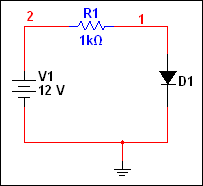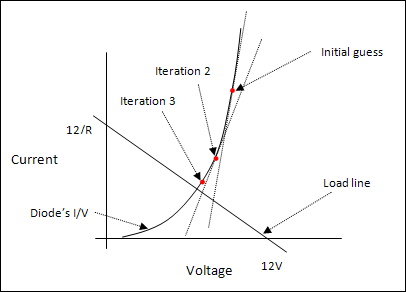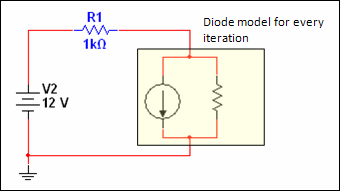# Linearization

## Multisim Help

Edition Date: February 2017
Part Number: 375482B-01
View Product InfoMultisim 14.0 and 14.0.1 HelpMultisim 14.1 HelpMultisim 14.2 Help

Non-linear elements pose a problem because the non-linear equations representing these elements cannot be solved directly as part of a system of equations. To address this problem, the simulator uses an iterative non-linear analysis technique called Newton-Raphson. To illustrate how this technique is used, consider the diode-resistor circuit shown below.The exact analytical solution to the circuit is found at the intersection of the load line and the diode's I/V line, as shown below.To find this intersection, the simulator begins by making a “guess” about the diode's voltage. The diode is then linearized about this guessed value using the derivative of the I/V curve. The linearization of the diode effectively models the diode as a resistor in parallel with a current source. This allows the simulator to use the Nodal Analysis and Matrix Solution process to find a solution.This first solution becomes the linearization value in the next iteration of the Newton-Raphson algorithm and the process continues until the difference in successive iterations becomes acceptably small. When this occurs, the iteration cycle is considered complete and the circuit solution is said to converge.

The example cited above is a very basic case of a non-linear circuit; the circuit contains only one non-linear element and there is continuity in the element's input-output curve and its derivative. In practice, the simulator has to deal with many non-linear elements and potential discontinuities in their input-output curves. As the number of non-linear elements increases, especially the number of elements with poor continuity characteristics, the chance for non-convergence likewise increases.

Convergence is one of the major hurdles in simulating non-linear circuits.

Convergence Criteria

For every voltage node, the following must be satisfied:

|V1 - V2| < tol

where,

V1 is the value of the present iteration
V2 is the value of the previous iteration
tol is the tolerance defined as RELTOL * Max(|V1|, |V2|) + VNTOL
where RELTOL and VNTOL are simulator options.

For every voltage-defined branch (the branch contains either a voltage source or an inductor), the following must be satisfied:

|I1 - I2| < tol

where,

I1 is the value of the present iteration
I2 is the value of the previous iteration
tol is the tolerance defined as RELTOL * Max(|I1|, |I2|) + ABSTOL
where RELTOL and ABSTOL are simulator options.

Related Information